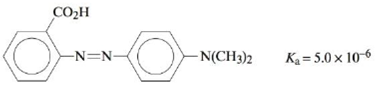# Methyl red has the following structure: It undergoes a color change from red to yellow as a solution gets more basic. Calculate an approximate pH range for which methyl red is useful. What is the color change and the pH at the color change when a weak acid is titrated with a strong base using methyl red as an indicator? What is the color change and the pH at the color change when a weak base is titrated with a strong acid using methyl red as an indicator? For which of these two types of titrations is methyl red a possible indicator?### Chemistry: An Atoms First Approach

2nd Edition
Steven S. Zumdahl + 1 other
Publisher: Cengage Learning
ISBN: 9781305079243

#### Solutions

Chapter
Section### Chemistry: An Atoms First Approach

2nd Edition
Steven S. Zumdahl + 1 other
Publisher: Cengage Learning
ISBN: 9781305079243
Chapter 14, Problem 70E
Textbook Problem
18 views

## Methyl red has the following structure:It undergoes a color change from red to yellow as a solution gets more basic. Calculate an approximate pH range for which methyl red is useful. What is the color change and the pH at the color change when a weak acid is titrated with a strong base using methyl red as an indicator? What is the color change and the pH at the color change when a weak base is titrated with a strong acid using methyl red as an indicator? For which of these two types of titrations is methyl red a possible indicator?

Interpretation Introduction

Interpretation: The color change of methyl red is given. The color change and the pH at the color change when a weak acid is titrated with a strong base using Methyl red as an indicator; the color change and the pH at the color change when a weak base is titrated with a strong acid using Methyl red as an indicator and the type of indicator for which Methyl red is used as a possible indicator is to be stated.

Concept introduction: The acid-base indicators show the color change with change in pH of the solution.

To determine: The color change and the pH at the color change when a weak acid is titrated with a strong base using Methyl red as an indicator; the color change and the pH at the color change when a weak base is titrated with a strong acid using Methyl red as an indicator and the type of indicator for which Methyl red is used as a possible indicator is to be stated.

### Explanation of Solution

Explanation

The pH range for which Methyl red is useful is 4.3-6.3_ .

Given

The value of pKa of Methyl red is 5.0×106 .

An indicator is a weak acid that remains in equilibrium with its conjugate base as,

HIn(aq)+H2O(l)H3O+(aq)+In(aq)

The pH change for the above reaction is given as,

pH=pKa+log[In][HIn] (1)

The indicators show a change in color in pKa range of ±1 . It means that the solution will retain their color till the time there is presence of at least 10% amount of other species. The value of pH=pKa+1 when the concentration of salt is ten times greater than acid while pH=pKa1 when the concentration of acid is ten times greater than the salt.

The relationship between pKa and Ka is given as,

pKa=logKa

Where,

• Ka is the dissociation constant of an acid.

Substitute the value of Ka in the above equation.

pKa=logKa=log(5.0×106)=5.30

Therefore, using the relation pH=pKa±1 , the value of pH comes out to be 4.3-6.3_ .

The pH range for which Methyl red is useful is 4.3-6.3_ .

Explanation

The pH of the solution when a weak acid is titrated with a strong base is 6.3_ and the color change is from red to yellow.

The dissociation of any indicator is shown as,

HIn(aq)+H2O(l)H3O+(aq)+In(aq)

The value of. Ka is given as,

Ka=[H3O+][In][HIn]

When a weak acid is titrated with strong base, the solution will be more basic therefore, the ratio of [In][HIn] is equal to 101

### Still sussing out bartleby?

Check out a sample textbook solution.

See a sample solution

#### The Solution to Your Study Problems

Bartleby provides explanations to thousands of textbook problems written by our experts, many with advanced degrees!

Get Started

Find more solutions based on key concepts
The volume 0.250 L could also be expressed as mL.

Introductory Chemistry: A Foundation

In what ways do melting glaciers become a problem?

Fundamentals of Physical Geography

When choosing a beverage, one should __________. a. read the label carefully, especially noting the number of s...

Nutrition: Concepts and Controversies - Standalone book (MindTap Course List)

How many centimeters are there in a kilometer?

An Introduction to Physical Science

What is marasmus?

Introduction to General, Organic and Biochemistry

What are sister chromatids?

Human Heredity: Principles and Issues (MindTap Course List)

What is a joint?

Human Biology (MindTap Course List)

What is the principal function of vitamin K in the human body?

General, Organic, and Biological Chemistry

Can the magnitude of a vector have a negative value? Explain.

Physics for Scientists and Engineers, Technology Update (No access codes included)